# Lesson 3 official topic

There are only two hard things in Computer Science: cache invalidation and naming things.
Phil Karlton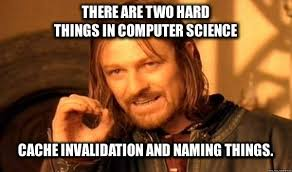Personally, I think it’s just an “accumulator” type variable that is accumulating the results as they pass through “layers” i.e., computations for layer1, activations (aka “non linearity”,) and computations for layer2.

For conciseness, the same variable is being used and since it is being returned as a “result”, it’s also called “res” (for result). But then again, it’s also accumulating a ‘residual’ value before being returned as the result. So it could represent either, or both?

But this is all conjecture on my part. Only the authors of the above mentioned code can clarify what their true intent was/is.

HTH

3 Likes

I use “res” for “result” in pretty much all my code. “residual” wouldn’t fit here because the residual is the difference between two things (e.g. targets vs predictions).

4 Likes

I am following the course in CA. Thus, I am usually one of two videos behind.

FYI, if you are downloading and training the HuggingFace Pets example [on google colab], “train.ipynb,” you get an error on the “untar_data()” method. It is because, in fastai 2.6.3, the function is moved to fastai.data.external.

I found the easy method to fix the “train.ipynb” is to add these (from the fastbook) line on the top cell:

``````
! [ -e /content ] && pip install -Uqq fastbook
import fastbook
fastbook.setup_book()
from fastbook import *
``````
2 Likes

In this lesson, when Jeremy creates a neural net in Excel with Titanic data what is the “shape” of this net? At first I thought it had two layers, but the parameters in each “layer” seem independent of each other.

Is this neural network instead comprised of two nodes within one layer? Would appreciate any context here - thanks!

In this exact screenshot (as the subtitle mentions), there’s no layers(neural network) yet. It’s a simple linear regression of the form `y = wx + b`.

As you can see in the pic, there’s a data table (input data rows) and parameters (that’s learnt by the excel solver), the SUMPRODUCT formula runs the whole linear regression in one sweep. (product of each data row(x1…xn) with parameters(w1…wn) = x1w1+…+xnwn, the results of each row product then being summed up).
Notice that the bias/constant term (b) is also converted into an extra column with just 1s which gets multiplied with Const (therefore, b*1), so no need for the extra addition separately.

1 Like

Understood that without a ReLU-non-linearity a stack of linear layers can do no more than a single linear equation, but I’m still interested in the question “What shape would this be?”

Considering just what is shown in the image: 15 rows, and P as 16th letter, 1 layer…
could this be said to have an equivalent shape… [15, 16, 1] ?

…no more than a linear transformation (function, mapping…). They are a bit different concepts. Note, for example, that a matrix expresses a linear transformation; yet it’s not a linear equation.

1 Like

If you download the file and play around, it might be a lot more clearer. There’s no matrix multiplication happening on this sheet (linear) of the excel file yet. I don’t have excel, but I’ll load it up in Google sheets and can try to walk through it a bit.

• There are 10 parameters (including the bias), so w1…w10.
• Now, for a SUMPRODUCT(w, x) to work, X would also need 10 elements (x1…x10).
• If you open the file you’ll see that we have 10 inputs (hence x1…x10) for each data row.
• Now, each row is being multplied to the params, then summed up (w1*x1+…) to get a single number as a ‘linear’ result (in the same row as the data column)
• You can consider this similar to a dot product of input vector  and params vector 
• This is done individually for each row to get the ‘linear’ result. If you open the excel file, you’ll find that there are 712 input rows(from 4 to 715). The formula for sumproduct is simply copied over this many times in the linear column.
• No matrix multiplications done yet in this sheet, for that you’d have to look into the ‘mmult’ sheet.

So,

• 10 input multiplied elementwise by 10 parameters elementwise, then summed up
• done for each row, hence 712 times gives us the Linear column
• loss is then calculated for each row, 712 times that gives us the Loss column
• finally all the loss is averaged

Here’s an example of one cell AC7, the 4th input row multplied to params to get the 4th linear loss item. The input and params are highlighted when editing the formula.

Hope this helps.4 Likes

I’m getting great results with convnext, it may end up becoming my new “go-to” (replacing resnets & efficientnets) for image classification tasks. Thanks to jeremy for the awesome write up comparing these new image model archs!

4 Likes

That’s great to hear James. I’m also def. using it as my default model going forward, esp. the `convnext_tiny` arch.

3 Likes

Very basic question: how do you make single predictions on the learner created during the notebook run? Calling predict fails with some error due to the resulting tensor being rank 0.

``````ex3 = tensor(Image.open(threes)).view(28*28).float()
ex3.shape, ex3

(torch.Size(),
tensor([  0.,   0.,   0.,   0.,   0.,   0.,   0.,   0.,   0.,   0.,   0.,   0.,
0.,   0.,   0.,   0.,   0.,   0.,   0.,   0.,   0.,   0.,   0.,   0.,
0.,   0.,   0.,   0.,   0.,   0.,   0.,   0.,   0.,   0.,   0.,   0.,....

learn.predict(ex3)

...
IndexError: too many indices for tensor of dimension 0
``````

I can work around the error by creating a test_dl and using get_preds instead, specifically altering the reorder parameter to false. The following works:

``````dl_ex3 = learn.dls.test_dl([ex3], batch_size=1)
preds,targs = learn.get_preds(dl=dl_ex3, act = torch.sigmoid, reorder=False)
``````
1 Like

Not sure if this will be useful. I’m a newbie taking this opportunity to challenge myself and learn.
Presuming you get “threes” from…

``````path = untar_data(URLs.MNIST_SAMPLE)
Path.BASE_PATH = path
path.ls()
(path/'train').ls()
threes = (path/'train'/'3').ls().sorted()
Image.open(threes).to_thumb(256,256)
``````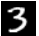I don’t see MNIST mentioned in YouTube-Transcript for the 2022 Lesson 3**, so for a simple notebook to experiment with I’ll use my copy of “Is it a bird” from Lesson 1

``````im3 = Image.open(threes)
t3 = tensor(im3)
v3 = t3.view(28*28)
ex3 = v3.float()
threes, im3.shape, t3.shape, v3.shape, ex3.shape
``````

(Path(‘train/3/10000.png’),
(28, 28),
torch.Size([28, 28]),
torch.Size(),
torch.Size())

I’m first curious why you change the shape from 28,28 to 784? (I’m not sure whether it matters)
Then splicing that into the bird predict…

``````is_bird,_,probs = learn.predict(ex3)
print(f"This is a: {is_bird}.")
print(f"Probability it's a bird: {probs:.4f}")
``````

This is a: bird.
Probability it’s a bird: 0.6929

while its unsure whether its a bird, i didn’t get an error.
So sorry, without being able to reproduce your error thats as far as I can go.

P.S…
**I do see MNIST in 2020 Lesson 3 Transcript, so could you clarify which lesson you were watching?

It’s in chapter 4 of the book.

1 Like

The book chapter 4 example utilizes a linear layer with 28*28 inputs. The image matrix is concatenated into a vector for feeding the neural network.

Check out the book, it is a great resource for a deeper diver.

1 Like

New didactic and methodic ideas - like them very much - still a bit rough in execution - but discovers amazing new territory to approach neural networks - deep learning … well done!

I tried the chap 4 notebook but there’s a difference in result with shuffle=True or shuffle=False in training dataset. With shuffle=True, losses are converging and accuracy is increaing while with shuffle=False, they are not. Setting shuffle=True seems correct but it doesn’t seem to work. Does anyone have any idea why? Here’s experiment: Why ‘shuffle’ prevents trainng? | fastpages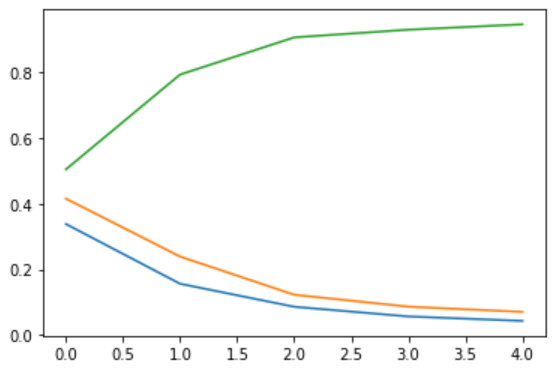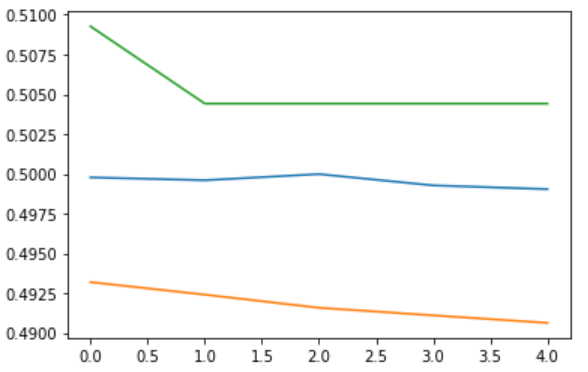1 Like

If I add unsqueeze ‘y’, shuffle works OK, where y.ndim 1 vs 0. Does anyone know why ‘y’ has to be 2 dimensional here at shuffle? Or where to look to find this out in DataLoader()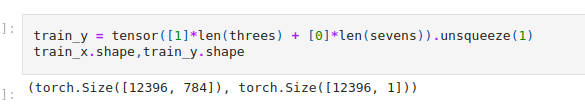Here’s the example of the above problem, Should ‘y’ be 2 dimensional in DataLoader()? | fastpages

I just made a single layer and double layer of RELUnet that I used in predicting the survived column of the Titanic dataset. It is the python version of what @jeremy did with excel. The model performed so well and achieved a 77% accuracy on the test set (which it had not seen before). I tested this on kaggle myself. The link to the notebook can be found here

I hope anyone finds it interactive and I’m open to suggestions.

Thanks.

1 Like

Hi,
When doing the excel exercise, Jeremy connects the two layers of the nnet by adding the outputs. For nnet I thought one would use the output of the first layer as the input to the second layer.
Am I missing something?
Thank you!

Edit: Is each of the “layers” actually one neuron, with one set of weights? Thus, together they actually form one layer - not two layers?

1 Like